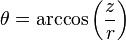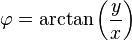## Dimensions and different coordinate sytems

The number of figures necessary for specifying the position of a point is called the dimension of the space.

For a two dimensional space it is sufficient to use two numbers (x, y) : one specifying the position on a right ot left scale and the other number giving the position on the up- and down scale. (x,y) are often referred to as the cartesian coordinates of a point.

Alternatively one can use polar coordinates where one uses the angle between an arrow pointing at the point and the length of the arrow (r, φ).

These are related by x=r cos(φ) and y =rsin(φ)

In three-dimensional space, our world for example, consequently three numbers are necessary. (x, y, z)

An alternative is the spherical-polar coordinates involving the radius, the azimuthal angle (φ) and the the declination (θ).These coordinates can be found from the cartesian coordinates by using the formulas(Pythagorean theorem in three dimensions)Annons## Om mattelararen

Licentiate of Philosophy in atomic Physics Master of Science in Physics
Detta inlägg publicerades i Algebra, Geometri, matematik 1c, Uncategorized och märktes , . Bokmärk permalänken.

### En kommentar till Dimensions and different coordinate sytems

1. Pingback: Polar rose | iMath